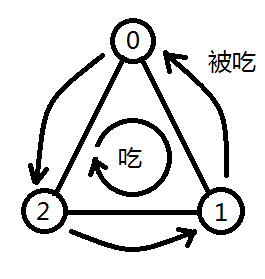# 并查集

2016-05-19 00:48 86 查看

并查集实现不相交集合的元素查询和集合合并。

并查集的实现大概是一个森林的结构，节点属性father为节点的父亲，其中 x.father == x 作为根节点的标志，（也可以指向x.father == -1等等作为标志）,各元素用所在树的根节点代表，查询一个元素相当于返回这个元素所在树的根节点，合并两个集合是把一个集合的根节点作为另一个集合的根节点的孩子，即合并setA, setB 的主要操作为  setA.root.father = setB.root;

```#define MAX 10005
int father[MAX];
void makeset(int n)
{
for (int i=1;i<=n;i++) father[i] = i;
}
int find(int x)
{
return x==father[x]?father[x]:father[x]=find(father[x]);
}
void unionset(int x,int y)  //合并y所在集合到x所在集合
{
father[find(y)] = find(x);
}```

void makeset(int n);

int find(int x)

void unionset(int x,int y)

1、poj1611 The Suspect

```#include <cstdio>
#define MAX 30005
int father[MAX], num[MAX];
void makeset(int n)
{
for (int i=0;i<n;i++)
{
father[i] = i;
num[i] = 1;
}
}
int find(int x)
{
return father[x]==x?father[x]:father[x]=find(father[x]);
}
void unionset(int x,int y)
{
x = find(x);
y = find(y);
if (x==y) return ;
father[y] = x;
num[x] += num[y];
}
int main(void)
{
int n,m,k,i,a,b;
while (~scanf("%d%d",&n,&m),n|m)
{
makeset(n);
while (m--)
{
scanf("%d",&k);
if (k) scanf("%d",&a);
for (i=1;i<k;i++)
{
scanf("%d",&b);
unionset(a,b);
}
}
printf("%d\n",num[find(0)]);
}
return 0;
}```

2、poj2524 Ubiquitous Religions

```#include <cstdio>
#define MAX 50005
int father[MAX],num[MAX],diff;
void makeset(int n)
{
for (int i=1;i<=n;i++)
{
father[i] = i;
num[i] = 1;
}
}
int find(int x)
{
if (x!=father[x]) father[x] = find(father[x]);
return father[x];
}
void unionset(int x,int y)
{
x = find(x);
y = find(y);
if (x==y) return ;
if (num[x] > num[y])//把num小的集合并到num大的集合，貌似可以减少树的高度。
{
father[y] = x;
num[x] += num[y];
}
else
{
father[x] = y;
num[y] += num[y];
}
diff--;
}
int main(void)
{
int n,m,cas = 1;
while (~scanf("%d%d",&n,&m),n|m)
{
diff = n;
makeset(n);
for (int i=0;i<m;i++)
{
int a,b;
scanf("%d%d",&a,&b);
unionset(a,b);
}
printf("Case %d: %d\n",cas++,diff);
}
return 0;
}```

3.、poj1182 食物链每次遇到x,y，如果它们在不同集合，那么它们还没有关系，一定不是谎言，然后把第两个集合并到第一个集合上，并根据操作是1或2更新第二个集合每个元素的关系域。

p.relation = (y.relation - x.relation + d + 2 )%3; //(1)

a.relation = (a.relation + a.father.relation)%3; //(2)

现在我们来看它们已经在同一集合的情况。这时，它们必然已经有关系了，那么就要判断语句给出的关系是否正确。还是假设输入为d,x,y，如果

(relation[x]-relation[y]+3)%3 != d-1代表是假话。只要加一个计数器计假话数就好了。这样我们就得到了整个题目的算法。
最后要注意的是，在真正实现的时候，(2)那里并不需要真的把整棵树更新，相反我们可以在查找函数上做功夫，使得每次查找的时候就更新查找那个结点的关系域就行了。

最后的最后，本题数据有点坑爹！只能一次输入，改成多次输入会WA。

#include <cstdio>
#include <cstdlib>
#include <cstring>
#include <algorithm>
#define MAX 50005
using namespace std;
int father[MAX], relation[MAX];//仍然是用数组表示
void makeset(int n)
{
for (int i=1;i<=n;i++)
{
father[i] = i;
relation[i] = 0;
}
}
int find(int x)
{
if (x!=father[x])
{
int temp = father[x];
father[x] = find(father[x]);
relation[x] = (relation[x]+relation[temp])%3; //在查找的时候更新所需结点的关系域
}
return father[x];
}
void unionset(int x,int y, int d)
{
int p = find(x), q = find(y);
father[p] = q;
relation[p] = (relation[y]-relation[x]+d+2)%3; //这里只更新p的关系域，当查找到p的子树种节点的时候，查找函数会帮忙更新那个结点
}
int main(void)
{
#ifdef EMO
freopen("in.txt","r",stdin);
freopen("out.txt","w",stdout);
#endif
int n,k,lie=0;
scanf("%d%d",&n,&k);
makeset(n);
while (k--)
{
int d,x,y,p,q;
scanf("%d%d%d",&d,&x,&y);
if (x>n || y>n) {lie++; continue;}
p = find(x);
q = find(y);
if (p!=q) unionset(x,y,d);
else if ((relation[x]-relation[y]+3)%3!=d-1)
{
lie++;
}
}
printf("%d\n",lie);
return 0;
}

http://www.cnblogs.com/cherish_yimi/archive/2009/10/11/1580839.html http://blog.csdn.net/lalor/article/details/7388805